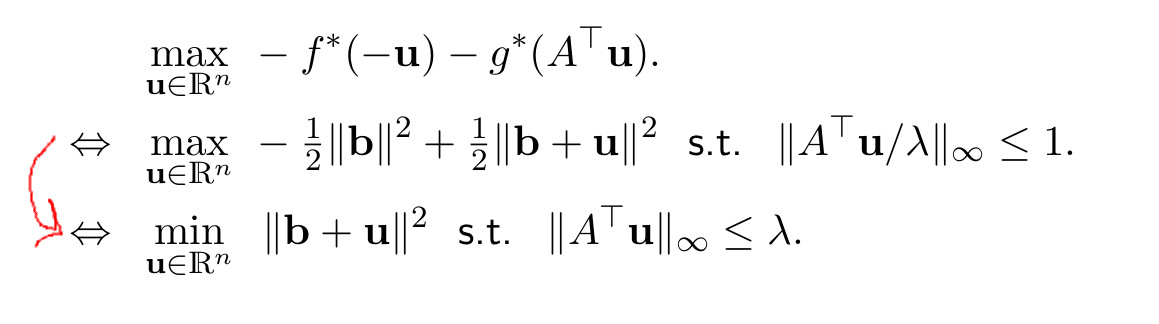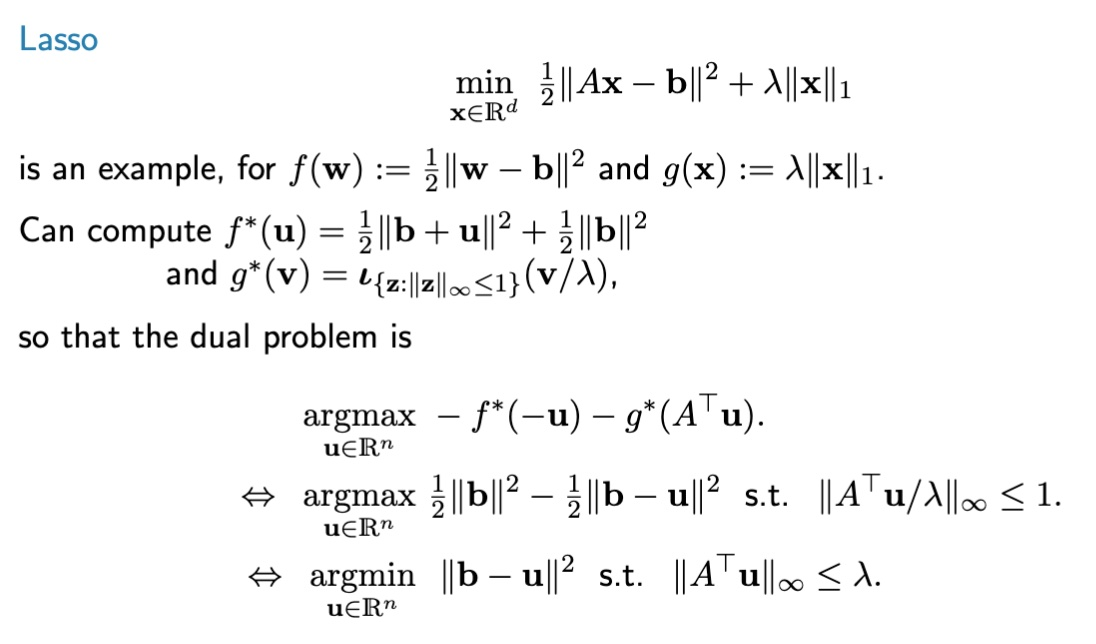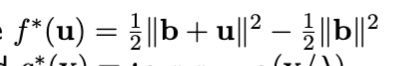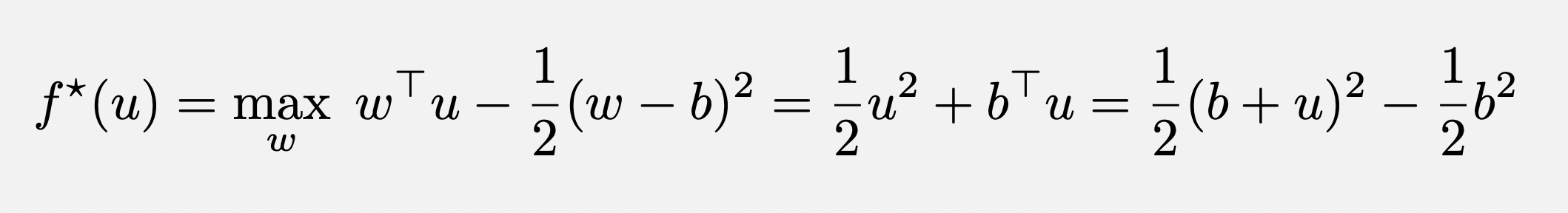### lecture 10, slide 7

Hi,

In slide 7 of lecture 10, I don't understand how we can get from max to min in the last two lines.Top comment

I think there are errors on the slide, and it should look like this:Hi, it looks to me like something is going wrong on this slide. I think the definition of f*(x) on this slide is inconsistent with the course's definition of the Fenchel conjugate (I get something else). I'll double-check and get back to you. Thanks!

Top comment

I think there are errors on the slide, and it should look like this:Yes, that answers my question. However, I have two more questions:

The penultimate line should be

$$argmax \{-\frac{1}{2}||b||^2 - \frac{1}{2}||b-u||^2 \}$$

right?

Also, would it be possible to have a more detailed derivation of the conjugate of $$f(w)=\frac{1}{2}||w-b||^2$$ ?

Hi Sepideh,

Ah, no the penultimate line is correct, but the conjugate should have beenMy derivation looked like this (where I set the derivative to zero to find $$w$$)